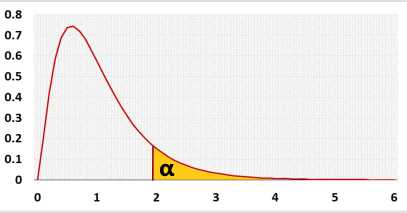# F sample size calculatorPower analysis

## Information

Calculate the sample size to gain the required test power and draw a power analysis chart.
Use this calculator for the following test:
F test For variances calculator

### F Distribution## R Code

The following R code should produce the same results